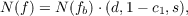#### Vol. 52, No. 1, 1974

 Download this articleFor screen For printingRecent Issues Vol. 325: 1 Vol. 324: 1  2 Vol. 323: 1  2 Vol. 322: 1  2 Vol. 321: 1  2 Vol. 320: 1  2 Vol. 319: 1  2 Vol. 318: 1  2Online Archive Volume: Issue:The Journal Subscriptions Editorial Board Officers Contacts Submission Guidelines Submission Form Policies for Authors ISSN: 1945-5844 (e-only) ISSN: 0030-8730 (print) Special Issues Author Index To Appear Other MSP Journals
On the Nielsen number of a fiber map

### Dennis McGavran and Jingyal Pak

Vol. 52 (1974), No. 1, 149–156
##### Abstract

Suppose 𝒯 = {E,π,B,F} is a fiber space such that 0 π1(F) π1(E)i# π1(B)π# 0 is exact. Suppose also that the above fundamental groups are abelian. If f : E E is a fiber preserving map such that f\$(α) = α if and only if α = 0, then it is shown that R(f) = R(f) R(fb) where R(h) is the Reidemeister number of the map h.

A product formula for the Nielsen number of a fiber map which holds under certain conditions was introduced by R. Brown. Let 𝒯 = {E,π,L,(p,q),s1} be a principal s1-bundle over the lens space L(p,q), where 𝒯 is determined by [ff] [L(p,q),cp] H2(L(p,q),z) zp. Let f : E E be a fiber preserving map such that fb#(1) = c2,f#(lp) = c1, where 1 generates π1(s1) z and lp generates π1(L(p,q)) zp. Then the Nielsen numbers of the maps involved satisfywhere d = (j,p) and s = j∕p(c1 c2).

Primary: 55C20
Secondary: 55F05
##### Milestones